## 1 Clojure with Static Typing

The popularity of dynamically-typed languages in software development, combined with a recognition that types often improve programmer productivity, software reliability, and performance, has led to the recent development of a wide variety of optional and gradual type systems aimed at checking existing programs written in existing languages. These include TypeScript  and Flow  for JavaScript, Hack  for PHP, and mypy  for Python among the optional systems, and Typed Racket , Reticulated Python , and GradualTalk  among gradually-typed systems.Footnote 1

One key lesson of these systems, indeed a lesson known to early developers of optional type systems such as StrongTalk, is that type systems for existing languages must be designed to work with the features and idioms of the target language. Often this takes the form of a core language, be it of functions or classes and objects, together with extensions to handle distinctive language features.

We synthesize these lessons to present Typed Clojure, an optional type system for Clojure. Clojure is a dynamically typed language in the Lisp family—built on the Java Virtual Machine (JVM)—which has recently gained popularity as an alternative JVM language. It offers the flexibility of a Lisp dialect, including macros, emphasizes a functional style via immutable data structures, and provides interoperability with existing Java code, allowing programmers to use existing Java libraries without leaving Clojure. Since its initial release in 2007, Clojure has been widely adopted for “backend” development in places where its support for parallelism, functional programming, and Lisp-influenced abstraction is desired on the JVM. As a result, there is an extensive base of existing untyped programs whose developers can benefit from Typed Clojure, an experience we discuss in this paper.

Since Clojure is a language in the Lisp family, we apply the lessons of Typed Racket, an existing gradual type system for Racket, to the core of Typed Clojure, consisting of an extended $$\lambda$$-calculus over a variety of base types shared between all Lisp systems. Furthermore, Typed Racket’s occurrence typing has proved necessary for type checking realistic Clojure programs.

However, Clojure goes beyond Racket in many ways, requiring several new type system features which we detail in this paper. Most significantly, Clojure supports, and Clojure developers use, multimethods to structure their code in extensible fashion. Furthermore, since Clojure is an untyped language, dispatch within multimethods is determined by application of dynamic predicates to argument values. Fortunately, the dynamic dispatch used by multimethods has surprising symmetry with the conditional dispatch handled by occurrence typing. Typed Clojure is therefore able to effectively handle complex and highly dynamic dispatch as present in existing Clojure programs.

But multimethods are not the only Clojure feature crucial to type checking existing programs. As a language built on the Java Virtual Machine, Clojure provides flexible and transparent access to existing Java libraries, and Clojure/Java interoperation is found in almost every significant Clojure code base. Typed Clojure therefore builds in an understanding of the Java type system and handles interoperation appropriately. Notably, null is a distinct type in Typed Clojure, designed to automatically rule out null-pointer exceptions.

An example of these features is given in Fig. 1. Here, the pname multimethod dispatches on the class of the argument—for Strings, the first method implementation is called, for Files, the second. The String method calls a File constructor, returning a non-nil File instance—the getName method on File requires a non-nil target, returning a nilable type.

Finally, flexible, high-performance immutable dictionaries are the most common Clojure data structure. Simply treating them as uniformly-typed key-value mappings would be insufficient for existing programs and programming styles. Instead, Typed Clojure provides a flexible heterogenous map type, in which specific entries can be specified.

While these features may seem disparate, they are unified in important ways. First, they leverage the type system mechanisms inherited from Typed Racket—multimethods when using dispatch via predicates, Java interoperation for handling null tests, and heterogenous maps using union types and reasoning about subcomponents of data. Second, they are crucial features for handling Clojure code in practice. Typed Clojure’s use in real Clojure deployments would not be possible without effective handling of these three Clojure features.

Our main contributions are as follows:

1. 1.

We motivate and describe Typed Clojure, an optional type system for Clojure that understands existing Clojure idioms.

2. 2.

We present a sound formal model for three crucial type system features: multi-methods, Java interoperability, and heterogenous maps.

3. 3.

We evaluate the use of Typed Clojure features on existing Typed Clojure code, including both open source and in-house systems.

The remainder of this paper begins with an example-driven presentation of the main type system features in Sect. 2. We then incrementally present a core calculus for Typed Clojure covering all of these features together in Sect. 3 and prove type soundness (Sect. 4). We then present an empirical analysis of significant code bases written in core.typed —the full implementation of Typed Clojure—in Sect. 5. Finally, we discuss related work and conclude.

## 2 Overview of Typed Clojure

We now begin a tour of the central features of Typed Clojure, beginning with Clojure itself. Our presentation uses the full Typed Clojure system to illustrate key type system ideas,Footnote 2 before studying the core features in detail in Sect. 3.

### 2.1 Clojure

Clojure  is a Lisp that runs on the Java Virtual Machine with support for concurrent programming and immutable data structures in a mostly-functional style. Clojure provides easy interoperation with existing Java libraries, with Java values being like any other Clojure value. However, this smooth interoperability comes at the cost of pervasive null, which leads to the possibility of null pointer exceptions—a drawback we address in Typed Clojure.

### 2.2 Typed Clojure

A simple one-argument function greet is annotated with ann to take and return strings.

Providing nil (exactly Java’s null) is a static type error—nil is not a string.

Unions. To allow nil, we use ad-hoc unions (nil and false are logically false).

Typed Clojure prevents well-typed code from dereferencing nil.

Flow Analysis. Occurrence typing  models type-based control flow. In greetings, a branch ensures repeat is never passed nil.

Removing the branch is a static type error—repeat cannot be passed nil.

### 2.3 Java Interoperability

Clojure can interact with Java constructors, methods, and fields. This program calls the getParent on a constructed File instance, returning a nullable string.

Typed Clojure can integrate with the Clojure compiler to avoid expensive reflective calls like getParent, however if a specific overload cannot be found based on the surrounding static context, a type error is thrown.

Function arguments default to Any, which is similar to a union of all types. Ascribing a parameter type allows Typed Clojure to find a specific method.

The conditional guards from dereferencing nil, and—as before—removing it is a static type error, as typed code could possibly dereference nil.

Typed Clojure rejects programs that assume methods cannot return nil.

Method targets can never be nil. Typed Clojure also prevents passing nil as Java method or constructor arguments by default—this restriction can be adjusted per method.

In contrast, JVM invariants guarantee constructors return non-null.Footnote 3

### 2.4 Multimethods

Multimethods are a kind of extensible function—combining a dispatch function with one or more methods—widely used to define Clojure operations.

Value-based Dispatch. This simple multimethod takes a keyword (Kw) and says hello in different languages.

When invoked, the arguments are first supplied to the dispatch function—identity—yielding a dispatch value. A method is then chosen based on the dispatch value, to which the arguments are then passed to return a value.

For example, (hi :en) evaluates to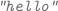—it executes the :en method because (= (identity :en) :en) is true and (= (identity :en) :fr) is false.

Dispatching based on literal values enables certain forms of method definition, but this is only part of the story for multimethod dispatch.

Class-based Dispatch. For class values, multimethods can choose methods based on subclassing relationships. Recall the multimethod from Fig. 1. The dispatch function class dictates whether the String or File method is chosen. The multimethod dispatch rules use isa?, a hybrid predicate which is both a subclassing check for classes and an equality check for other values.

The current dispatch value and—in turn—each method’s associated dispatch value is supplied to isa?. If exactly one method returns true, it is chosen. For example, the call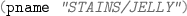picks the String method because (isa? String String) is true, and (isa? String File) is not.

### 2.5 Heterogeneous Hash-Maps

The most common way to represent compound data in Clojure are immutable hash-maps, typicially with keyword keys. Keywords double as functions that look themselves up in a map, or return nil if absent.

HMap types describe the most common usages of keyword-keyed maps.

This says :en and :fr are known entries mapped to strings, and the map is fully specified—that is, no other entries exist—by :complete? being true.

HMap types default to partial specification, with ’{:en Str :fr Str} abbreviating (HMap :mandatory {:en Str, :fr Str}).

HMaps in Practice. The next example is extracted from a production system at CircleCI, a company with a large production Typed Clojure system (Sect. 5.2 presents a case study and empirical result from this code base).

As EncKeyPair is fully specified, we remove extra keys like :priv via dissoc, which returns a new map that is the first argument without the entry named by the second argument. Notice removing dissoc causes a type error.

### 2.6 HMaps and Multimethods, Joined at the Hip

HMaps and multimethods are the primary ways for representing and dispatching on data respectively, and so are intrinsically linked. As type system designers, we must search for a compositional approach that can anticipate any combination of these features.

Thankfully, occurrence typing, originally designed for reasoning about if tests, provides the compositional approach we need. By extending the system with a handful of rules based on HMaps and other functions, we can automatically cover both easy cases and those that compose rules in arbitrary ways.

Futhermore, this approach extends to multimethod dispatch by reusing occurrence typing’s approach to conditionals and encoding a small number of rules to handle the isa?-based dispatch. In practice, conditional-based control flow typing extends to multimethod dispatch, and vice-versa.

We first demonstrate a very common, simple dispatch style, then move on to deeper structural dispatching where occurrence typing’s compositionality shines.

HMaps and Unions. Partially specified HMap’s with a common dispatch key combine naturally with ad-hoc unions. An Order is one of three kinds of HMaps.

The :Meal entry is common to each HMap, always mapped to a known keyword singleton type. It’s natural to dispatch on the class of an instance—it’s similarly natural to dispatch on a known entry like :Meal.

The :combo method is verified to only structurally recur on Orders. This is achieved because we learn the argument o must be of type ’{:Meal :combo} since (isa? (:Meal o) :combo) is true. Combining this with the fact that o is an Order eliminates possibility of :lunch and :dinner orders, simplifying o to ’{:Meal ’:combo :meal1 Order :meal2 Order} which contains appropriate arguments for both recursive calls.

Nested Dispatch. A more exotic dispatch mechanism for desserts might be on the class of the :desserts key. If the result is a number, then we know the :desserts key is a number, otherwise the input is a :combo meal. We have already seen dispatch on class and on keywords in isolation—occurrence typing automatically understands control flow that combines its simple building blocks.

The first method has dispatch value Long, a subtype of Int, and the second method has nil, the sentinel value for a failed map lookup. In practice, :lunch and :dinner meals will dispatch to the Long method, but Typed Clojure infers a slightly more general type due to the definition of :combo meals.

In the Long method, Typed Clojure learns that its argument is at least of type ’{:desserts Long}—since (isa? (class (:desserts o)) Long) must be true. Here the :desserts entry must be present and mapped to a Long—even in a :combo meal, which does not specify :desserts as present or absent.

In the nil method, (isa? (class (:desserts o)) nil) must be true—which implies (class (:desserts o)) is nil. Since lookups on missing keys return nil, either

• o has a :desserts entry to nil, like :desserts nil, or

• o is missing a :desserts entry.

We can express this type with the :absent-keys HMap option

This eliminates non-:combo meals since their ’{:desserts Int} type does not agree with this new information (because :desserts is neither nil or absent).

From Multiple to Arbitrary Dispatch. Clojure multimethod dispatch, and Typed Clojure’s handling of it, goes even further, supporting dispatch on multiple arguments via vectors. Dispatch on multiple arguments is beyond the scope of this paper, but the same intuition applies—adding support for multiple dispatch admits arbitrary combinations and nestings of it and previous dispatch rules.

## 3 A Formal Model of $$\lambda _{TC}$$

After demonstrating the core features of Typed Clojure, we link them together in a formal model called $$\lambda _{TC}$$. Building on occurrence typing, we incrementally add each novel feature of Typed Clojure to the formalism, interleaving presentation of syntax, typing rules, operational semantics, and subtyping.

### 3.1 Core Type System

We start with a review of occurrence typing , the foundation of $$\lambda _{TC}$$ .

Expressions. Syntax is given in Fig. 2. Expressions $$e_{}$$ include variables $$x_{}$$, values $$v_{}$$, applications, abstractions, conditionals, and let expressions. All binding forms introduce fresh variables—a subtle but important point since our type environments are not simply dictionaries. Values include booleans $$b_{}$$, $${\mathsf {nil}}$$, class literals $$C_{}$$, keywords $$k_{}$$, integers $$n_{}$$, constants $$c_{}$$, and strings $$s_{}$$. Lexical closures $$[\rho _{}, \lambda x_{}^{\tau _{}} . e_{} ]_{{\mathsf {c}}}$$ close value environments $$\rho _{}$$—which map bindings to values—over functions.

Types. Types $$\sigma _{}$$ or $$\tau _{}$$ include the top type $$\mathop {\mathbf{\top }}\nolimits$$, untagged unions ($$\mathop {\mathbf{\bigcup }}\nolimits \ \overrightarrow{\tau _{}}$$), singletons $${(\mathop {\mathbf{Val}}\nolimits l_{}){}}$$, and class instances $$C_{}$$. We abbreviate the classes $$\mathop {\mathbf{Boolean}}\nolimits$$ to B, $$\mathop {\mathbf{Keyword}}\nolimits$$ to K, $$\mathop {\mathbf{Nat}}\nolimits$$ to N, $$\mathop {\mathbf{String}}\nolimits$$ to S, and $$\mathop {\mathbf{File}}\nolimits$$ to F. We also abbreviate the types $$(\mathop {\mathbf{\bigcup }}\nolimits )$$ to $$\mathop {\mathbf{\bot }}\nolimits$$ , ($$\mathop {\mathbf{Val}}\nolimits {{\mathsf {nil}}}$$) to nil, ($$\mathop {\mathbf{Val}}\nolimits {{\mathsf {true}}}$$) to $$\mathop {\mathbf{true}}\nolimits$$, and ($$\mathop {\mathbf{Val}}\nolimits {{\mathsf {false}}}$$) to $$\mathop {\mathbf{false}}\nolimits$$. The difference between the types ($$\mathop {\mathbf{Val}}\nolimits C_{}$$) and $$C_{}$$ is subtle. The former is inhabited by class literals like $$\mathop {\mathbf{K}}\nolimits$$ and the result of $$(class {}\ {\mathsf {\mathbin {:}a}})$$—the latter by instances of classes, like a keyword literal $${\mathsf {\mathbin {:}a}}$$, an instance of the type K. Function types $${{x_{} {:} \sigma _{}} \xrightarrow [o_{}]{\psi _{} | \psi _{}} \tau _{}}$$ contain latent (terminology from ) propositions $$\psi _{}$$, object $$o_{}$$, and return type $$\tau _{}$$, which may refer to the function argument $$x_{}$$. They are instantiated with the actual object of the argument in applications.

Objects. Each expression is associated with a symbolic representation called an object. For example, variable $$m$$ has object $$m$$;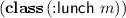has object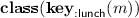; and 42 has the empty object $$\emptyset _{}$$ since it is unimportant in our system. Figure 2 gives the syntax for objects $$o_{}$$—non-empty objects $$\pi _{}(x_{})$$ combine of a root variable $$x_{}$$ and a path $$\pi _{}$$, which consists of a possibly-empty sequence of path elements ($$pe_{}$$) applied right-to-left from the root variable. We use two path elements—$$\mathbf{{class} } _{}$$ and $${\mathbf{key }}_{k}$$—representing the results of calling class and looking up a keyword k, respectively.

Propositions with a Logical System. In standard type systems, association lists often track the types of variables, like in LC-Let and LC-Local.

Occurrence typing instead pairs logical formulas, that can reason about arbitrary non-empty objects, with a proof system. The logical statement $${\sigma _{}}_{x_{}}$$ says variable x is of type $$\sigma _{}$$.

In T0-Local, $${ \Gamma {} \vdash {\tau _{}}_{x_{}} }$$ appeals to the proof system to solve for $$\tau _{}$$.

We further extend logical statements to propositional logic. Figure 2 describes the syntax for propositions $$\psi _{}$$, consisting of positive and negative type propositions about non-empty objects—$${\tau _{}}_{\pi _{}(x_{})}$$ and $${\overline{\tau _{}}}_{\pi _{}(x_{})}$$ respectively—the latter pronounced “the object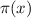is not of type $$\tau _{}$$”. The other propositions are standard logical connectives: implications, conjunctions, disjunctions, and the trivial (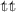) and impossible (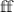) propositions. The full proof system judgement $${ \Gamma {} \vdash \psi _{} }$$ says proposition environment $$\Gamma$$ proves proposition $$\psi _{}$$.

Each expression is associated with two propositions—when expression $$e_{1}$$ is in test position like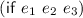, the type system extracts $$e_{1}$$’s ‘then’ and ‘else’ proposition to check $$e_{2}$$ and $$e_{3}$$ respectively. For example, in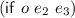we learn variable $$o$$ is true in $$e_{2}$$ via $$o$$’s ‘then’ proposition $${\overline{\mathop {\mathbf{(\cup \ \mathop {\mathbf{nil}}\nolimits \ \mathop {\mathbf{false}}\nolimits )}}\nolimits {}}}_{o}$$, and that $$o$$ is false in $$e_{3}$$ via $$o$$’s ‘else’ proposition $${\mathop {\mathbf{(\cup \ \mathop {\mathbf{nil}}\nolimits \ \mathop {\mathbf{false}}\nolimits )}}\nolimits {}}_{o}$$.

To illustrate, recall Example 8. The parameter $$o$$ is of type $$\mathop {\mathbf{Order}}\nolimits$$, written $${\mathop {\mathbf{Order}}\nolimits }_{o}$$ as a proposition. In the $${{\mathsf {\mathbin {:}combo}}}$$ method, we know $${({\mathsf {\mathbin {:}Meal}}\ o)}$$ is $${{\mathsf {\mathbin {:}combo}}}$$, based on multimethod dispatch rules. This is written $${(\mathop {\mathbf{Val}}\nolimits {\mathsf {\mathbin {:}combo}} )}_{{\mathbf{key }}_{{\mathsf {\mathbin {:}Meal}} } (o)}$$, pronounced “the $${{\mathsf {\mathbin {:}Meal}}}$$ path of variable $$o$$ is of type ($$\mathop {\mathbf{Val}}\nolimits {\mathsf {\mathbin {:}combo}}$$)”.

To attain the type of $$o$$, we must solve for $$\tau _{}$$ in $${ \Gamma {} \vdash {\tau _{}}_{o} }$$, under proposition environment $$\Gamma {} = {{{\mathop {\mathbf{Order}}\nolimits }_{o}}, {{(\mathop {\mathbf{Val}}\nolimits {\mathsf {\mathbin {:}combo}} )}_{{\mathbf{key }}_{{\mathsf {\mathbin {:}Meal}} } (o)}}}$$ which deduces $$\tau _{}$$ to be a $${\mathsf {\mathbin {:}combo}}$$ meal. The logical system combines pieces of type information to deduce more accurate types for lexical bindings—this is explained in Sect. 3.6.

Typing Judgment. We formalize our system following Tobin-Hochstadt and Felleisen . The typing judgment $${\Gamma \vdash {e_{} \Rightarrow e'_{} \mathbin {:} \tau _{}}\ ;\ {\psi _{}}_+ | {\psi _{}}_- \ ;\ o_{}}$$ says expression $$e_{}$$ rewrites to $$e'_{}$$, which is of type $$\tau _{}$$ in the proposition environment $$\Gamma {}$$, with ‘then’ proposition $${\psi _{}}_+$$, ‘else’ proposition $${\psi _{}}_-$$ and object $$o_{}$$.

We write $$\Gamma \vdash {e_{} \Rightarrow e'_{} \mathbin {:} \tau _{}}$$ to mean $$\Gamma \vdash {e_{} \Rightarrow e'_{} \mathbin {:} \tau _{}}\ ;\ {\psi '_{}}_+ | {\psi '_{}}_- \ ;\ o'_{}$$ for some $${\psi '_{}}_+$$, $${\psi '_{}}_-$$ and $$o'_{}$$, and abbreviate self rewriting judgements $$\Gamma \vdash {e_{} \Rightarrow e_{} \mathbin {:} \tau _{}}\ ;\ {\psi _{}}_+ | {\psi _{}}_- \ ;\ o_{}$$ to $$\Gamma \vdash {e_{} \mathbin {:} \tau _{}}\ ;\ {\psi _{}}_+ | {\psi _{}}_- \ ;\ o_{}$$.

Typing Rules. The core typing rules are given as Fig. 3. We introduce the interesting rules with the complement number predicate as a running example.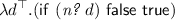(1)

The lambda rule T-Abs introduces $${\sigma _{}}_{x_{}}$$ = $${\mathop {\mathbf{\top }}\nolimits }_{d}$$ to check the body. With $$\Gamma$$ = $${\mathop {\mathbf{\top }}\nolimits }_{d}$$, T-If first checks the test $$e_{1}$$ = $$(n? {}\ d)$$ via the T-App rule, with three steps.

First, in T-App the operator $$e_{}$$ = n? is checked with T-Const, which uses $${\delta }_{\tau _{}}$$ (Fig. 7, dynamic semantics in the supplemental material) to type constants. n? is a predicate over numbers, and class returns its argument’s class.

Resuming $$(n? {}\ d)$$, in T-App the operand $$e'_{}$$ = $$d$$ is checked with T-Local as

\begin{aligned} \Gamma {} \vdash {d \mathbin {:} \mathop {\mathbf{\top }}\nolimits }\ ;\ {\overline{\mathop {\mathbf{(\cup \ \mathop {\mathbf{nil}}\nolimits \ \mathop {\mathbf{false}}\nolimits )}}\nolimits }}_{d} | {\mathop {\mathbf{(\cup \ \mathop {\mathbf{nil}}\nolimits \ \mathop {\mathbf{false}}\nolimits )}}\nolimits }_{d} \ ;\ d \end{aligned}
(2)

which encodes the type, proposition, and object information about variables. The proposition $${\overline{\mathop {\mathbf{(\cup \ \mathop {\mathbf{nil}}\nolimits \ \mathop {\mathbf{false}}\nolimits )}}\nolimits }}_{d}$$ says “it is not the case that variable $$d$$ is of type $$\mathop {\mathbf{(\cup \ \mathop {\mathbf{nil}}\nolimits \ \mathop {\mathbf{false}}\nolimits )}}\nolimits$$”; $${\mathop {\mathbf{(\cup \ \mathop {\mathbf{nil}}\nolimits \ \mathop {\mathbf{false}}\nolimits )}}\nolimits }_{d}$$ says “$$d$$ is of type $$\mathop {\mathbf{(\cup \ \mathop {\mathbf{nil}}\nolimits \ \mathop {\mathbf{false}}\nolimits )}}\nolimits$$”.

Finally, the T-App rule substitutes the operand’s object $$o'_{}$$ for the parameter $$x_{}$$ in the latent type, propositions, and object. The proposition $${\mathop {\mathbf{N}}\nolimits {}}_{d}$$ says “$$d$$ is of type $$\mathop {\mathbf{N}}\nolimits$$ ”; $${\overline{\mathop {\mathbf{N}}\nolimits {}}}_{d}$$ says “it is not the case that $$d$$ is of type $$\mathop {\mathbf{N}}\nolimits$$ ”. The object $$d$$ is the symbolic representation of what the expression $$d$$ evaluates to.

\begin{aligned} \Gamma {} \vdash {(n? {}\ d) \mathbin {:} \mathop {\mathbf{B}}\nolimits {}}\ ;\ {\mathop {\mathbf{N}}\nolimits {}}_{d} | {\overline{\mathop {\mathbf{N}}\nolimits {}}}_{d} \ ;\ \emptyset _{} \end{aligned}
(3)

To demonstrate, the ‘then’ proposition—in T-App $${\psi _{}}_+ [o'_{} / x_{}]$$—substitutes the latent ‘then’ proposition of $${\delta }_{\tau _{}} {}(n? {})$$ with $$d$$, giving $${\mathop {\mathbf{N}}\nolimits {}}_{x_{}} [d / x_{}]$$ = $${\mathop {\mathbf{N}}\nolimits {}}_{d}$$.

To check the branches of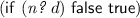, T-If introduces $${\psi _{1}}_+$$ = $${\mathop {\mathbf{N}}\nolimits {}}_{d}$$ to check $$e_{2}$$ = $${\mathsf {false}}$$, and $${\psi _{1}}_-$$ = $${\overline{\mathop {\mathbf{N}}\nolimits {}}}_{d}$$ to check $$e_{3}$$ = $${\mathsf {true}}$$. The branches are first checked with T-False and T-True respectively, the T-Subsume premises $$\Gamma {}, {{\psi _{}}_+} \vdash {\psi '_{}}_+$$ and $$\Gamma {}, {{\psi _{}}_-} \vdash {\psi '_{}}_-$$ allow us to pick compatible propositions for both branches.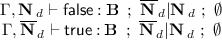Finally T-Abs assigns a type to the overall function: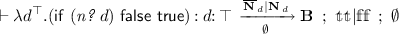Subtyping. Figure 4 presents subtyping as a reflexive and transitive relation with top type $$\mathop {\mathbf{\top }}\nolimits$$. Singleton types are instances of their respective classes—boolean singleton types are of type $$\mathop {\mathbf{B}}\nolimits$$ , class literals are instances of $$\mathop {\mathbf{Class}}\nolimits$$ and keywords are instances of $$\mathop {\mathbf{K}}\nolimits$$ . Instances of classes $$C_{}$$ are subtypes of $$\mathop {\mathbf{Object}}\nolimits$$ . Function types are subtypes of $$\mathop {\mathbf{Fn}}\nolimits$$ . All types except for $$\mathop {\mathbf{nil}}\nolimits$$ are subtypes of $$\mathop {\mathbf{Object}}\nolimits$$ , so $$\mathop {\mathbf{\top }}\nolimits$$ is similar to ($$\mathop {\mathbf{\bigcup }}\nolimits \ \mathop {\mathbf{nil}}\nolimits \ \mathop {\mathbf{Object}}\nolimits$$). Function subtyping is contravariant left of the arrow—latent propositions, object and result type are covariant. Subtyping for untagged unions is standard.

Operational Semantics. We define the dynamic semantics for $$\lambda _{TC}$$ in a big-step style using an environment, following . We include both errors and a $$wrong$$ value, which is provably ruled out by the type system. The main judgment is $$\rho _{} \vdash e_{} \Downarrow \alpha _{}$$ which states that $$e_{}$$ evaluates to answer $$\alpha _{}$$ in environment $$\rho _{}$$. We chose to omit the core rules (included in supplemental material) however a notable difference is $${\mathsf {nil}}$$ is a false value, which affects the semantics of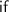(Fig. 5).

### 3.2 Java Interoperability

We present Java interoperability in a restricted setting without class inheritance, overloading or Java Generics. We extend the syntax in Fig. 6 with Java field lookups and calls to methods and constructors. To prevent ambiguity between zero-argument methods and fields, we use Clojure’s primitive “dot” syntax: field accesses are written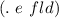and method calls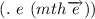.

In Example 1,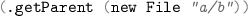translates to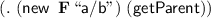(4)

But both the constructor and method are unresolved. We introduce non-reflective expressions for specifying exact Java overloads.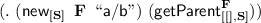(5)

From the left, the one-argument constructor for $$\mathop {\mathbf{F}}\nolimits$$ takes a $$\mathop {\mathbf{S}}\nolimits$$, and the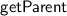method of $$\mathop {\mathbf{F}}\nolimits$$ takes zero arguments and returns a $$\mathop {\mathbf{S}}\nolimits$$.

We now walk through this conversion.

Constructors. First we check and convert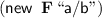to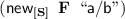. The T-New typing rule checks and rewrites constructors. To checkwe first resolve the constructor overload in the class table—there is at most one to simplify presentation. With $$C_{1}$$ = $$\mathop {\mathbf{S}}\nolimits$$, we convert to a nilable type the argument with $$\tau _{1}$$ = ($$\mathop {\mathbf{\bigcup }}\nolimits \ \mathop {\mathbf{nil}}\nolimits \ \mathop {\mathbf{S}}\nolimits$$) and type check $${{\mathsf {a/b}}}"$$ against $$\tau _{1}$$. Typed Clojure defaults to allowing non-nilable arguments, but this can be overridden, so we model the more general case. The return Java type $$\mathop {\mathbf{F}}\nolimits$$ is converted to a non-nil Typed Clojure type $$\tau _{}$$ = $$\mathop {\mathbf{F}}\nolimits$$ for the return type, and the propositions say constructors can never be false—constructors can never produce the internal boolean value that Clojure uses for $${\mathsf {false}}$$, or $${\mathsf {nil}}$$. Finally, the constructor rewrites to.

Methods. Next we convert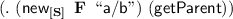to the non-reflective expression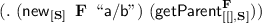. The T-Method rule for unresolved methods checks. We verify the target type $$\sigma _{}$$ = $$\mathop {\mathbf{F}}\nolimits$$ is non-nil by T-New. The overload is chosen from the class table based on $$C_{1}$$ = $$\mathop {\mathbf{F}}\nolimits$$—there is at most one. The nilable return type $$\tau _{}$$ = ($$\mathop {\mathbf{\bigcup }}\nolimits \ \mathop {\mathbf{nil}}\nolimits \ \mathop {\mathbf{S}}\nolimits$$) is given, and the entire expression rewrites to expression 5.

The T-Field rule (Fig. 6) is like T-Method, but without arguments.

The evaluation rules B-Field, B-New and B-Method (Fig. 6) simply evaluate their arguments and call the relevant JVM operation, which we do not model—Sect. 4 states our exact assumptions. There are no evaluation rules for reflective Java interoperability, since there are no typing rules that rewrite to reflective calls.

### 3.3 Multimethod Preliminaries: isa?

We now consider the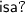operation, a core part of the multimethod dispatch mechanism. Recalling the examples in Sect. 2.4,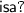is a subclassing test for classes, but otherwise is an equality test. The T-IsA rule uses $${{\mathsf {IsAProps}}}$$ (Fig. 8), a metafunction which produces the propositions forexpressions.

To demonstrate the first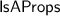case, the expression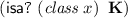is true if $$x_{}$$ is a keyword, otherwise false. When checked with T-IsA, the object of the left subexpression $$o_{}$$ = $$\mathbf{{class} } _{}(x_{})$$ (which starts with the $$\mathbf{{class} } _{}$$ path element) and the type of the right subexpression $$\tau _{}$$ = ($$\mathop {\mathbf{Val}}\nolimits \mathop {\mathbf{K}}\nolimits$$) (a singleton class type) together trigger the first $${{\mathsf {IsAProps}}}$$ case $${{\mathsf {IsAProps}}} (\mathbf{{class} } _{}(x_{}), (\mathop {\mathbf{Val}}\nolimits \mathop {\mathbf{K}}\nolimits )) = {{\mathop {\mathbf{K}}\nolimits }_{x_{}} | {\overline{\mathop {\mathbf{K}}\nolimits }}_{x_{}} }$$, giving propositions that correspond to our informal description $${\psi _{}}_+ | {\psi _{}}_-$$ = $${\mathop {\mathbf{K}}\nolimits }_{x_{}} | {\overline{\mathop {\mathbf{K}}\nolimits }}_{x_{}}$$.

The second $${{\mathsf {IsAProps}}}$$ case captures the simple equality mode for non-class singleton types. For example, the expression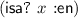produces true when $$x_{}$$ evaluates to $${\mathsf {\mathbin {:}en}}$$, otherwise it produces false. Using T-IsA, it has the propositions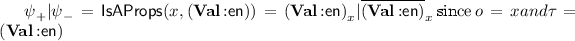. The side condition on the second $${{\mathsf {IsAProps}}}$$ case ensures we are in equality mode—if $$x_{}$$ can possibly be a class in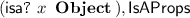uses its conservative default case, since if $$x_{}$$ is a class literal, subclassing mode could be triggered. Capture-avoiding substitution of objects $$[o_{} / x_{}]$$ used in this case erases propositions that would otherwise have $$\emptyset _{}$$ substituted in for their objects—it is defined in the appendix.

The operational behavior ofis given by B-IsA (Fig. 8). $${{\mathsf {IsA}}}$$ explicitly handles classes in the second case.

### 3.4 Multimethods

Figure 8 presents immutable multimethods without default methods to ease presentation. Figure 9 translates the mutable Example 4 to $$\lambda _{TC}$$ .

To check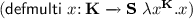, we note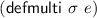creates a multimethod with interface type $$\sigma _{}$$, and dispatch function $$e_{}$$ of type $$\sigma {'}_{}$$, producing a value of type ($$\mathop {\mathbf{Multi}}\nolimits$$ $$\sigma _{}$$ $$\sigma {'}_{}$$). The T-DefMulti typing rule checks the dispatch function, and verifies both the interface and dispatch type’s domain agree. Our example checks with $$\tau _{}$$ = $$\mathop {\mathbf{K}}\nolimits$$, interface type $$\sigma _{}$$ = $${x_{} {:} \mathop {\mathbf{K}}\nolimits } \xrightarrow \ {\mathop {\mathbf{S}}\nolimits }$$, dispatch function type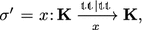and overall type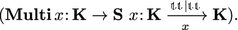Next, we show how to check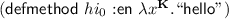. The expression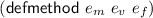creates a new multimethod that extends multimethod $$e_{m}$$’s dispatch table, mapping dispatch value $$e_{v}$$ to method $$e_{f}$$. The T-DefMulti typing rule checks $$e_{m}$$ is a multimethod with dispatch function type $$\tau _{d}$$, then calculates the extra information we know based on the current dispatch value $${\psi ''_{}}_+$$, which is assumed when checking the method body. Our example checks with $$e_{m}$$ being of type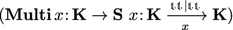with $$o'_{}$$ = $$x_{}$$ (from below the arrow on the right argument of the previous type) and $$\tau _{v}$$ = ($$\mathop {\mathbf{Val}}\nolimits {\mathsf {\mathbin {:}en}}$$). Then $${\psi ''_{}}_+$$ = $${(\mathop {\mathbf{Val}}\nolimits {\mathsf {\mathbin {:}en}} )}_{x_{}}$$ from $${{\mathsf {IsAProps}}} (x_{}, (\mathop {\mathbf{Val}}\nolimits {\mathsf {\mathbin {:}en}} )) = {{(\mathop {\mathbf{Val}}\nolimits {\mathsf {\mathbin {:}en}} )}_{x_{}} | {\overline{(\mathop {\mathbf{Val}}\nolimits {\mathsf {\mathbin {:}en}} )}}_{x_{}} }$$ (see Sect. 3.3). Since $$\tau _{}$$ = $$\mathop {\mathbf{K}}\nolimits$$ , we check the method body with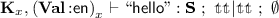. Finally from the interface type $$\tau _{m}$$, we know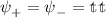, and $$o_{}$$ = $$\emptyset _{}$$, which also agrees with the method body, above. Notice the overall type of a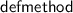is the same as its first subexpression $$e_{m}$$.

It is worth noting the lack of special typing rules for overlapping methods—each method is checked independently based on local type information.

Subtyping. Multimethods are functions, via S-PMultiFn, which says a multimethod can be upcast to its interface type. Multimethod call sites are then handled by T-App via T-Subsume. Other rules are given in Fig. 8.

Semantics. Multimethod definition semantics are also given in Fig. 8. B-DefMulti creates a multimethod with the given dispatch function and an empty dispatch table. B-DefMethod produces a new multimethod with an extended dispatch table.

The overall dispatch mechanism is summarised by B-BetaMulti. First the dispatch function $$v_{d}$$ is applied to the argument $$v'_{}$$ to obtain the dispatch value $$v_{e}$$. Based on $$v_{e}$$, the $${\mathsf {GM}}$$ metafunction (Fig. 8) extracts a method $$v_{f}$$ from the method table $$t_{}$$ and applies it to the original argument for the final result.

### 3.5 Precise Types for Heterogeneous Maps

Figure 10 presents heterogeneous map types. The type ($$\mathbf{HMap}^{\varepsilon }{\mathcal {M} _{}}\;{\mathcal {A} _{}}$$) contains $$\mathcal {M} _{}$$, a map of present entries (mapping keywords to types), $$\mathcal {A} _{}$$, a set of keyword keys that are known to be absent and tag $$\mathcal {E}_{}$$ which is either $$\mathcal {C}_{}$$ (“complete”) if the map is fully specified by $$\mathcal {M} _{}$$, and $$\mathcal {P}_{}$$ (“partial”) if there are unknown entries. The partially specified map of lunch in Example 6 is written ($$\mathop {\mathbf{HMap}}\nolimits ^{\mathcal {P}_{}}\{{(\mathop {\mathbf{Val}}\nolimits {\mathsf {\mathbin {:}en}} )}\ {\mathop {\mathbf{S}}\nolimits }, {{(\mathop {\mathbf{Val}}\nolimits {\mathsf {\mathbin {:}fr}} )}\ {\mathop {\mathbf{S}}\nolimits }}\}\ \{\} {}$$) (abbreviated $$\mathop {\mathbf{Lu}}\nolimits$$). The type of the fully specified map breakfast in Example 5 elides the absent entries, written ($$\mathop {\mathbf{HMap}}\nolimits ^{\mathcal {C}_{}}\{{(\mathop {\mathbf{Val}}\nolimits {\mathsf {\mathbin {:}en}} )}\ {\mathop {\mathbf{S}}\nolimits }, {{(\mathop {\mathbf{Val}}\nolimits {\mathsf {\mathbin {:}fr}} )}\ {\mathop {\mathbf{S}}\nolimits }}\}$$) (abbreviated $$\mathop {\mathbf{Bf}}\nolimits$$). To ease presentation, if an HMap has completeness tag $$\mathcal {C}_{}$$ then $$\mathcal {A} _{}$$ is elided and implicitly contains all keywords not in the domain of $$\mathcal {M} _{}$$—dissociating keys is not modelled, so the set of absent entries otherwise never grows. Keys cannot be both present and absent.

The metavariable $$m_{}$$ ranges over the runtime value of maps $$\{{\overrightarrow{{k_{}} \mapsto {v_{}}}}\}$$, usually written $$\{{\overrightarrow{{k_{}}\ {v_{}}}}\}$$. We only provide syntax for the empty map literal, however when convenient we abbreviate non-empty map literals to be a series of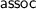operations on the empty map. We restrict lookup and extension to keyword keys.

How to Check. A mandatory lookup is checked by T-GetHMap.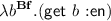The result type is $$\mathop {\mathbf{S}}\nolimits$$, and the return object is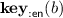. The object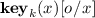is a symbolic representation for a keyword lookup of k in $$o_{}$$. The substitution for $$x_{}$$ handles the case where $$o_{}$$ is empty.

An absent lookup is checked by T-GetHMapAbsent.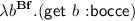The result type is $$\mathop {\mathbf{nil}}\nolimits$$—since $$\mathop {\mathbf{Bf}}\nolimits$$ is fully specified—with return object $${\mathbf{key }}_{{\mathsf {\mathbin {:}bocce}} } (b)$$.

A lookup that is not present or absent is checked by T-GetHMapPartialDefault.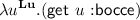The result type is $$\mathop {\mathbf{\top }}\nolimits$$—since $$\mathop {\mathbf{Lu}}\nolimits$$ has an unknown $${\mathsf {\mathbin {:}bocce}}$$ entry—with return object $${\mathbf{key }}_{{\mathsf {\mathbin {:}bocce}} } (u)$$. Notice propositions are erased once they enter a HMap type.

For presentational reasons, lookups on unions of HMaps are only supported in T-GetHMap and each element of the union must contain the relevant key.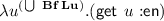The result type is $$\mathop {\mathbf{S}}\nolimits$$, and the return object is $${\mathbf{key }}_{{\mathsf {\mathbin {:}en}} } (u)$$. However, lookups of $${\mathsf {\mathbin {:}bocce}}$$ on ($$\mathop {\mathbf{\bigcup }}\nolimits \ \mathop {\mathbf{Bf}}\nolimits \mathop {\mathbf{Lu}}\nolimits$$) maps are unsupported. This restriction still allows us to check many of the examples in Sect. 2—in particular we can check Example 8, as $${\mathsf {\mathbin {:}Meal}}$$ is in common with both HMaps, but cannot check Example 9 because a $${\mathsf {\mathbin {:}combo}}$$ meal lacks a $${\mathsf {\mathbin {:}desserts}}$$ entry. Adding a rule to handle Example 9 is otherwise straightforward.

Extending a map with T-AssocHMap preserves its completeness.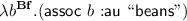The result type is $$(\mathop {\mathbf{HMap}}\nolimits ^{\mathcal {C}_{}}\{{(\mathop {\mathbf{Val}}\nolimits {\mathsf {\mathbin {:}en}} )}\ {\mathop {\mathbf{S}}\nolimits }, {{(\mathop {\mathbf{Val}}\nolimits {\mathsf {\mathbin {:}fr}} )}\ {\mathop {\mathbf{S}}\nolimits }}, {{(\mathop {\mathbf{Val}}\nolimits {\mathsf {\mathbin {:}au}} )}\ {\mathop {\mathbf{S}}\nolimits }}\} )$$, a complete map. T-AssocHMap also enforces $${k_{}} \not \in {\mathcal {A} _{}}$$ to prevent badly formed types.

Subtyping. Subtyping for HMaps designate $$\mathop {\mathbf{Map}}\nolimits$$ as a common supertype for all HMaps. S-HMap says that HMaps are subtypes if they agree on $$\mathcal {E}_{}$$, agree on mandatory entries with subtyping and at least cover the absent keys of the supertype. Complete maps are subtypes of partial maps as long as they agree on the mandatory entries of the partial map via subtyping (S-HMapP).

The semantics for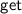andare straightforward.

### 3.6 Proof System

The occurrence typing proof system uses standard propositional logic, except for where nested information is combined. This is handled by L-Update:

It says under $$\Gamma$$ , if object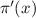is of type $$\tau _{}$$, and an extension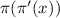is of possibly-negative type $$\nu _{}$$, then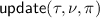is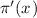’s type under $$\Gamma$$ .

Recall Example 8. Solving $${ {{\mathop {\mathbf{Order}}\nolimits }_{o}}, {{(\mathop {\mathbf{Val}}\nolimits {\mathsf {\mathbin {:}combo}} )}_{{\mathbf{key }}_{{\mathsf {\mathbin {:}Meal}} } (o)}} \vdash {\tau _{}}_{o} }$$ uses L-Update, where $$\pi _{}$$ = $$\epsilon$$ and $$\pi '_{}$$ = [$${\mathbf{key }}_{{\mathsf {\mathbin {:}Meal}} }$$].

$$\Gamma {} \vdash {{\mathsf {update}} {({\mathop {\mathbf{Order}}\nolimits }, {(\mathop {\mathbf{Val}}\nolimits {\mathsf {\mathbin {:}combo}} )}, {[{{\mathbf{key }}_{{\mathsf {\mathbin {:}Meal}} }}]})}}_{o}$$

Since $$\mathop {\mathbf{Order}}\nolimits$$ is a union of HMaps, we structurally recur on the first case of $${\mathsf {update}}$$ (Fig. 12), which preserves $$\pi _{}$$. Each initial recursion hits the first HMap case, since there is some $$\tau _{}$$ such that $${\mathcal {M} _{}}[{k_{}}] = {\tau _{}}$$ and $$\mathcal {E}_{}$$ accepts partial maps $$\mathcal {P}_{}$$.

To demonstrate, $${\mathsf {\mathbin {:}lunch}}$$ meals are handled by the first HMap case and update to ($$\mathop {\mathbf{HMap}}\nolimits ^{\mathcal {P}_{}}\mathcal {M} _{}[{(\mathop {\mathbf{Val}}\nolimits {\mathsf {\mathbin {:}Meal}} )} \mapsto {\sigma {'}_{}} ]\ \{\} {}$$) where $$\sigma {'}_{}$$ = $${\mathsf {update}}$$ ($$(\mathop {\mathbf{Val}}\nolimits {\mathsf {\mathbin {:}lunch}} )$$, $$(\mathop {\mathbf{Val}}\nolimits {\mathsf {\mathbin {:}combo}} )$$, $$\epsilon$$) and $$\mathcal {M} _{}$$ = $$\{{(\mathop {\mathbf{Val}}\nolimits {\mathsf {\mathbin {:}Meal}} )} \mapsto {(\mathop {\mathbf{Val}}\nolimits {\mathsf {\mathbin {:}lunch}} )},{{(\mathop {\mathbf{Val}}\nolimits {\mathsf {\mathbin {:}desserts}} )} \mapsto {\mathop {\mathbf{N}}\nolimits {}}}\}$$. $$\sigma {'}_{}$$ updates to $$\mathop {\mathbf{\bot }}\nolimits$$ via the penultimate $${\mathsf {update}}$$ case, because $${\mathsf {restrict}}$$ (($$\mathop {\mathbf{Val}}\nolimits {\mathsf {\mathbin {:}lunch}}$$), ($$\mathop {\mathbf{Val}}\nolimits {\mathsf {\mathbin {:}combo}}$$)) = $$\mathop {\mathbf{\bot }}\nolimits$$ by the first $${\mathsf {restrict}}$$ case. The same happens to $${\mathsf {\mathbin {:}dinner}}$$ meals, leaving just the $${\mathsf {\mathbin {:}combo}}$$ HMap.

In Example 9, $$\Gamma {} \vdash {{\mathsf {update}} {({\mathop {\mathbf{Order}}\nolimits }, {\mathop {\mathbf{Long}}\nolimits }, {[{\mathbf{{class} } _{}}, {{\mathbf{key }}_{{\mathsf {\mathbin {:}desserts}} }}]})}}_{o}$$ updates the argument in the $$\mathop {\mathbf{Long}}\nolimits$$ method. This recurs twice for each meal to handle the $$\mathbf{{class} } _{}$$ path element.

We describe the other $${\mathsf {update}}$$ cases. The first $$\mathbf{{class} } _{}$$ case updates to $$C_{}$$ if class returns ($$\mathop {\mathbf{Val}}\nolimits C_{}$$). The second $${\mathbf{key }}_{k_{}}$$ case detects contradictions in absent keys. The third $${\mathbf{key }}_{k_{}}$$ case updates unknown entries to be mapped to $$\tau _{}$$ or absent. The fourth $${\mathbf{key }}_{k_{}}$$ case updates unknown entries to be present when they do not overlap with $$\mathop {\mathbf{nil}}\nolimits$$ .

## 4 Metatheory

We prove type soundness following Tobin-Hochstadt and Felleisen . Our model is extended to include errors $${\mathsf {err}}$$ and a $$wrong$$ value, and we prove well-typed programs do not go wrong; this is therefore a stronger theorem than proved by Tobin-Hochstadt and Felleisen . Errors behave like Java exceptions—they can be thrown and propagate “upwards” in the evaluation rules ($${\mathsf {err}}$$ rules are deferred to the appendix).

Rather than modeling Java’s dynamic semantics, a task of daunting complexity, we instead make our assumptions about Java explicit. We concede that method and constructor calls may diverge or error, but assume they can never go wrong (other assumptions given in the supplemental material).

### Assumption 1

$$\mathbf{( }\mathsf{JVM}_{\mathsf{new}}\mathbf{). }$$ If $$\forall i.\ {v_{i}} = {C_{i}\ \{\overrightarrow{fld_{j} : v_{j}}\}}\ or\ {v_{i}}= {{{\mathsf {nil}}}}$$ and $$v_{i}$$ is consistent with $$\rho _{}$$ then either

• $${\mathsf {JVM}} _{{\mathsf {new}}} [C_{}, [\overrightarrow{C_{i}}], [\overrightarrow{v_{i}}]] = C_{}\ \{\overrightarrow{fld_{k} : v_{k}}\}$$ which is consistent with $$\rho _{}$$,

• $${\mathsf {JVM}} _{{\mathsf {new}}} [C_{}, [\overrightarrow{C_{i}}], [\overrightarrow{v_{i}}]] = {\mathsf {err}}$$, or

• $${\mathsf {JVM}} _{{\mathsf {new}}} [C_{}, [\overrightarrow{C_{i}}], [\overrightarrow{v_{i}}]]$$ is undefined.

For the purposes of our soundness proof, we require that all values are consistent. Consistency (defined in the supplemental material) states that the types of closures are well-scoped—they do not claim propositions about variables hidden in their closures.

We can now state our main lemma and soundness theorem. The metavariable $$\alpha _{}$$ ranges over $$v_{}$$, $${\mathsf {err}}$$ and $$wrong$$ . Proofs are deferred to the supplemental material.

### Lemma 1

If $$\Gamma {} \vdash {e'_{} \Rightarrow e_{} \mathbin {:} \tau _{}}\ ;\ {\psi _{}}_+ | {\psi _{}}_- \ ;\ o_{}$$, $${\rho _{}} \models {\Gamma {}}$$, $${\rho _{}}\ \text {is consistent}$$, and $$\rho _{} \vdash e_{} \Downarrow \alpha {}$$ then either

• $$\rho _{} \vdash e_{} \Downarrow v_{}$$ and all of the following hold:

1. 1.

either $$o_{}$$ = $$\emptyset _{}$$ or $$\rho _{} (o_{}) = v_{}$$,

2. 2.

either $${\mathsf {TrueVal}}(v_{})$$ and $${\rho _{}} \models {{\psi _{}}_+}$$ or $${\mathsf {FalseVal}}(v_{})$$ and $${\rho _{}} \models {{\psi _{}}_-}$$,

3. 3.

$$\vdash {v_{} \Rightarrow v_{} \mathbin {:} \tau _{}}\ ;\ {\psi '_{}}_+ | {\psi '_{}}_- \ ;\ o'_{}$$ for some $${\psi '_{}}_+$$, $${\psi '_{}}_-$$ and $$o'_{}$$, and

4. 4.

$${v_{}}\ \text {is consistent with}\ {\rho _{}}$$, or

• $$\rho _{} \vdash e_{} \Downarrow {\mathsf {err}} {}$$.

### Theorem 1

(Type Soundness). If $$\Gamma {} \vdash {e'_{} \Rightarrow e_{} \mathbin {:} \tau _{}}\ ;\ {\psi _{}}_+ | {\psi _{}}_- \ ;\ o_{}$$ and $$\rho _{} \vdash e_{} \Downarrow v_{}$$ then $$\vdash {v_{} \Rightarrow v_{} \mathbin {:} \tau _{}}\ ;\ {\psi '_{}}_+ | {\psi '_{}}_- \ ;\ o'_{}$$ for some $${\psi '_{}}_+$$, $${\psi '_{}}_-$$ and $$o'_{}$$.

## 5 Experience

Typed Clojure is implemented as core.typed  , which has seen wide usage.

### 5.1 Implementation

core.typed provides preliminary integration with the Clojure compilation pipeline, primarily to resolve Java interoperability.

The core.typed implementation extends this paper in several key areas to handle checking real Clojure code, including an implementation of Typed Racket’s variable-arity polymorphism , and support for other Clojure idioms like datatypes and protocols. There is no integration with Java Generics, so only Java 1.4-style erased types are “trusted” by core.typed. Casts are needed to recover the discarded information, which—for collections—are then tracked via Clojure’s universal sequence interface .

### 5.2 Evaluation

Throughout this paper, we have focused on three interrelated type system features: heterogenous maps, Java interoperability, and multimethods. Our hypothesis is that these features are widely used in existing Clojure programs in interconnecting ways, and that handling them as we have done is required to type check realistic Clojure programs.

To evaluate this hypothesis, we analyzed two existing core.typed code bases, one from the open-source community, and one from a company that uses core.typed in production. For our data gathering, we instrumented the core.typed type checker to record how often various features were used (summarized in Fig. 13).

feeds2imap. feeds2imapFootnote 4 is an open source library written in Typed Clojure. It provides an RSS reader using the javax.mail framework.

Of 11 typed namespaces containing 825 lines of code, there are 32 Java interactions. The majority are method calls, consisting of 20 (62 %) instance methods and 5 (16 %) static methods. The rest consists of 1 (3 %) static field access, and 6 (19 %) constructor calls—there are no instance field accesses.

There are 27 lookup operations on HMap types, of which 20 (74 %) resolve to mandatory entries, 6 (22 %) to optional entries, and 1 (4 %) is an unresolved lookup. No lookups involved fully specified maps.

From 93 def expressions in typed code, 52 (56 %) are checked, with a rate of 1 Java interaction for 1.6 checked top-level definitions, and 1 HMap lookup to 1.9 checked top-level definitions. That leaves 41 (44 %) unchecked vars, mainly due to partially complete porting to Typed Clojure, but in some cases due to unannotated third-party libraries.

No typed multimethods are defined or used. Of 18 total type aliases, 7 (39 %) contained one HMap type, and none contained unions of HMaps—on further inspection there was no HMap entry used to dictate control flow, often handled by multimethods. This is unusual in our experience, and is perhaps explained by feeds2imap mainly wrapping existing javax.mail functionality.

CircleCI. CircleCI  provides continuous integration services built with a mixture of open- and closed-source software. Typed Clojure was used at CircleCI in production systems for two years , maintaining 87 namespaces and 19,000 lines of code, an experience we summarise in Sect. 5.3.

The CircleCI code base contains 11 checked multimethods. All 11 dispatch functions are on a HMap key containing a keyword, in a similar style to Example 8. Correspondingly, all 89 methods are associated with a keyword dispatch value. The argument type was in all cases a single HMap type, however, rather than a union type. In our experience from porting other libraries, this is unusual.

Of 328 lookup operations on HMaps, 208 (64 %) resolve to mandatory keys, 70 (21 %) to optional keys, 20 (6 %) to absent keys, and 30 (9 %) lookups are unresolved. Of 95 total type aliases defined with defalias, 62 (65 %) involved one or more HMap types. Out of 105 Java interactions, 26 (25 %) are static methods, 36 (34 %) are instance methods, 38 (36 %) are constructors, and 5 (5 %) are static fields. 35 methods are overriden to return non-nil, and 1 method overridden to accept nil—suggesting that core.typed disallowing nil as a method argument by default is justified.

Of 464 checked top-level definitions (which consists of 57 defmethod calls and 407 def expressions), 1 HMap lookup occurs per 1.4 top-level definitions, and 1 Java interaction occurs every 4.4 top-level definitions.

From 1834 def expressions in typed code, only 407 (22 %) were checked. That leaves 1427 (78 %) which have unchecked definitions, either by an explicit :no-check annotation or tc-ignore to suppress type checking, or the warn-on-unannotated-vars option, which skips def expressions that lack expected types via ann. From a brief investigation, reasons include unannotated third-party libraries, work-in-progress conversions to Typed Clojure, unsupported Clojure idioms, and hard-to-check code.

Lessons. Based on our empirical survey, HMaps and Java interoperability support are vital features used on average more than once per typed function. Multimethods are less common in our case studies. The CircleCI code base contains only 26 multimethods total in 55,000 lines of mixed untyped-typed Clojure code, a low number in our experience.

### 5.3 Further Challenges

After a 2 year trial, the second case study decided to disabled type checking . They were supportive of the fundamental ideas presented in this paper, but primarily cited issues with the checker implementation in practice and would reconsider type checking if they were resolved. This is also supported by Fig. 13, where 78 % of def expressions are unchecked.

Performance. Rechecking files with transitive dependencies is expensive since all dependencies must be rechecked. We conjecture caching type state will significantly improve re-checking performance, though preserving static soundness in the context of arbitrary code reloading is a largely unexplored area.

Library Annotations. Annotations for external code are rarely available, so a large part of the untyped-typed porting process is reverse engineering libraries.

Unsupported Idioms. While the current set of features is vital to checking Clojure code, there is still much work to do. For example, common Clojure functions are often too polymorphic for the current implementation or theory to account for. The post-mortem  contains more details.

## 6 Related Work

Multimethods. Millstein  and collaborators present a sequence of systems [4, 5, 20] with statically-typed multimethods and modular type checking. In contrast to Typed Clojure, in these system methods declare the types of arguments that they expect which corresponds to exclusively using class as the dispatch function in Typed Clojure. However, Typed Clojure does not attempt to rule out failed dispatches.

Record Types. Row polymorphism [3, 12, 26], used in systems such as the OCaml object system, provides many of the features of HMap types, but defined using universally-quantified row variables. HMaps in Typed Clojure are instead designed to be used with subtyping, but nonetheless provide similar expressiveness, including the ability to require presence and absence of certain keys.

Dependent JavaScript  can track similar invariants as HMaps with types for JS objects. They must deal with mutable objects, they feature refinement types and strong updates to the heap to track changes to objects.

TeJaS , another type system for JavaScript, also supports similar HMaps, with the ability to record the presence and absence of entries, but lacks a compositional flow-checking approach like occurrence typing.

Typed Lua  has table types which track entries in a mutable Lua table. Typed Lua changes the dynamic semantics of Lua to accommodate mutability: Typed Lua raises a runtime error for lookups on missing keys—HMaps consider lookups on missing keys normal.

Java Interoperability in Statically Typed Languages. Scala  has nullable references for compatibility with Java. Programmers must manually check for null as in Java to avoid null-pointer exceptions.

Other Optional and Gradual Type Systems. Several other gradual type systems have been developed for existing dynamically-typed languages. Reticulated Python  is an experimental gradually typed system for Python, implemented as a source-to-source translation that inserts dynamic checks at language boundaries and supporting Python’s first-class object system. Clojure’s nominal classes avoids the need to support first-class object system in Typed Clojure, however HMaps offer an alternative to the structural objects offered by Reticulated. Similarly, Gradualtalk  offers gradual typing for Smalltalk, with nominal classes.

Optional types have been adopted in industry, including Hack , and Flow  and TypeScript , two extensions of JavaScript. These systems support limited forms of occurrence typing, and do not include the other features we present.

## 7 Conclusion

Optional type systems must be designed with close attention to the language that they are intended to work for. We have therefore designed Typed Clojure, an optionally-typed version of Clojure, with a type system that works with a wide variety of distinctive Clojure idioms and features. Although based on the foundation of Typed Racket’s occurrence typing approach, Typed Clojure both extends the fundamental control-flow based reasoning as well as applying it to handle seemingly unrelated features such as multi-methods. In addition, Typed Clojure supports crucial features such as heterogeneous maps and Java interoperability while integrating these features into the core type system. Not only are each of these features important in isolation to Clojure and Typed Clojure programmers, but they must fit together smoothly to ensure that existing untyped programs are easy to convert to Typed Clojure.

The result is a sound, expressive, and useful type system which, as implemented in core.typed with appropriate extensions, is suitable for typechecking a significant amount of existing Clojure programs. As a result, Typed Clojure is already successful: it is used in the Clojure community among both enthusiasts and professional programmers.

Our empirical analysis of existing Typed Clojure programs bears out our design choices. Multimethods, Java interoperation, and heterogeneous maps are indeed common in both Clojure and Typed Clojure, meaning that our type system must accommodate them. Furthermore, they are commonly used together, and the features of each are mutually reinforcing. Additionally, the choice to make Java’s null explicit in the type system is validated by the many Typed Clojure programs that specify non-nullable types.